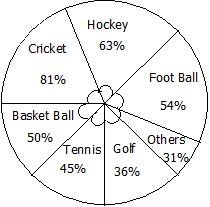# Percentage TNPSC Group 1 Questions

## Percentage MCQ Questions

13.

A number is increased by 10% and then decrease by 10%. Find the net decrease percent.

Group 1 - 2017

A.

0%

B.

1%

C.

2%

D.

3%

B. 1%

14.

The length of a rectangle is increased by 60%. By what percent would the width have to be decreased so as to maintain the same area

Group 2A - 2016

A.

37 1/2%

B.

60%

C.

75%

D.

120%

A. 37 1/2%

15.

If the length of a rectangle is decreased by 50 % and the breadth is increased by 80 % then the % change in the area of rectangle is

Group 2 - 2015

A.

decreased by 10%

B.

increased by 10 %

C.

decreased by 20 %

D.

increased by 20 %

A. decreased by 10%

16.

The circle-graph given here shows the spendings of a country on various sports during a particular year. Study the graph and answer the question.What percent of the total spending is spent on Tennis?

Group 2A - 2014

A.

12 1/2%

B.

22 1/2%

C.

25%

D.

45%

A. 12 1/2%

17.

If A's salary is 20% less than B's salary, by how much percent is B's salary more than A's?

Group 2A - 2014

A.

24%

B.

25%

C.

20%

D.

22%

B. 25%

18.

If 50% of (x-y) = 30% of (x + y) then what percent of x is y?

Group 2A - 2014

A.

25%

B.

50%

C.

75%

D.

100%